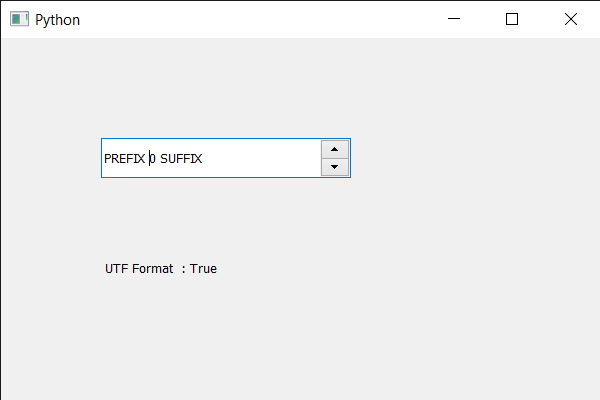Related Articles

# PyQt5 QSpinBox – Checking if the character is UTF-32 format

• Last Updated : 26 May, 2020

In this article we will see how we can check if the given character in the font of the spin box is in UTF-32 format. UTF-32 is a fixed-length encoding used to encode Unicode code points that uses exactly 32 bits per code point. UTF-32 is a fixed-length encoding, in contrast to all other Unicode transformation formats, which are variable-length encodings.

In order to do this we use `inFontUcs4` method with the spin box’s QFontMetrics object.

Syntax : font_metrics.inFontUcs4(unit_character)

Argument : It takes integer as argument

Return : It returns bool.

Below is the implementation

 `# importing libraries``from` `PyQt5.QtWidgets ``import` `*` `from` `PyQt5 ``import` `QtCore, QtGui``from` `PyQt5.QtGui ``import` `*` `from` `PyQt5.QtCore ``import` `*` `import` `sys`` ` ` ` `class` `Window(QMainWindow):`` ` `    ``def` `__init__(``self``):``        ``super``().__init__()`` ` `        ``# setting title``        ``self``.setWindowTitle(``"Python "``)`` ` `        ``# setting geometry``        ``self``.setGeometry(``100``, ``100``, ``600``, ``400``)`` ` `        ``# calling method``        ``self``.UiComponents()`` ` `        ``# showing all the widgets``        ``self``.show()`` ` `        ``# method for widgets``    ``def` `UiComponents(``self``):``        ``# creating spin box``        ``self``.spin ``=` `QSpinBox(``self``)`` ` `        ``# setting geometry to spin box``        ``self``.spin.setGeometry(``100``, ``100``, ``250``, ``40``)`` ` `        ``# setting range to the spin box``        ``self``.spin.setRange(``0``, ``999999``)`` ` `        ``# setting prefix to spin``        ``self``.spin.setPrefix(``"PREFIX "``)`` ` `        ``# setting suffix to spin``        ``self``.spin.setSuffix(``" SUFFIX"``)`` ` `        ``# creating a label``        ``label ``=` `QLabel(``self``)`` ` `        ``# making label multi line``        ``label.setWordWrap(``True``)`` ` `        ``# setting geometry to the label``        ``label.setGeometry(``100``, ``200``, ``300``, ``60``)`` ` `        ``# getting font metrics``        ``f_metrics ``=` `self``.spin.fontMetrics()`` ` `        ``# checking if the character is in the font``        ``check ``=` `f_metrics.inFontUcs4(``1``)`` ` `        ``# setting text to the label``        ``label.setText(``" UTF Format  : "` `+` `str``(check))`` ` ` ` `# create pyqt5 app``App ``=` `QApplication(sys.argv)`` ` `# create the instance of our Window``window ``=` `Window()`` ` `# start the app``sys.exit(App.``exec``())`

Output :Attention geek! Strengthen your foundations with the Python Programming Foundation Course and learn the basics.

To begin with, your interview preparations Enhance your Data Structures concepts with the Python DS Course. And to begin with your Machine Learning Journey, join the Machine Learning – Basic Level Course

My Personal Notes arrow_drop_up